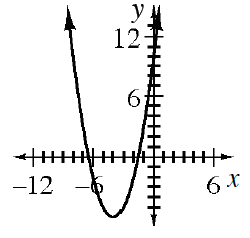### Home > INT2 > Chapter 11 > Lesson 11.2.3 > Problem11-86

11-86.

Rewrite $y = x^2 + 8x + 10$ in graphing form and sketch a graph of the function. Then name the vertex and the $y$-intercept.

Complete the square:
$y + 16 = (x + 4)^2 + 10$
$y = (x + 4)^2 - 6$

The vertex is the lowest point on the graph and the $y$ intercept is the point where $x = 0$.

Vertex $= (-4, -6)$
$y$ intercept $= (0, 10)$